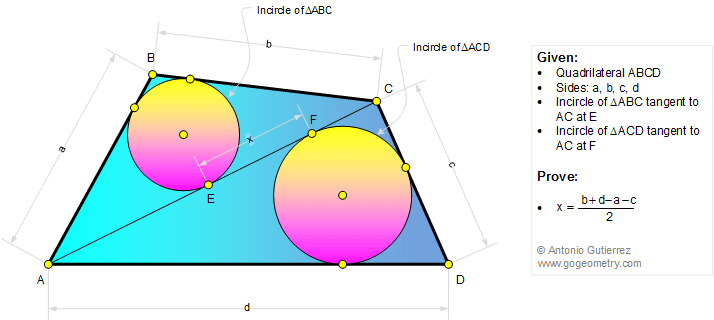# Geometry Problem 1308

### Quadrilateral, Diagonal, Triangle, Incircle, Tangent Line, Sides, Measurement

The figure below shows a quadrilateral ABCD with sides a, b, c, and d. The incircles of triangles ABC and ADC are tangent to AC at E, and F, respectively. Prove that EF = (b + d - a - c)/2.### Typography of problem 1308 in Motion using Mobile Apps, iPad

Click on the figure below.